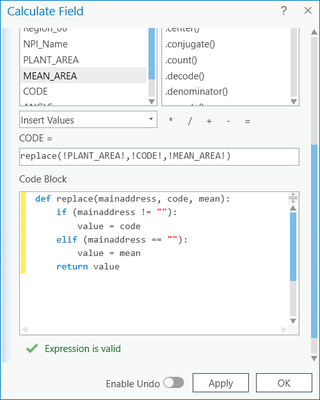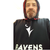# Add a fieldname instead of value in calculate field using Python

288
4
Jump to solution
02-10-2021 01:41 PMOccasional Contributor

I am trying to convert this VB script in a model from ArcMap (10.7.1) to a model in ArcGIS Pro (3.7).

Calculating a field ADD1, and  using values from a field ADD2:

Dim MainAddress
If Not [ADD1]= "" Then
MainAddress = MainAddress
Else
MainAddress = [ADD2]
End if

______

I attempted this in the ArcGIS Pro codeblock: and was unable to replace the value with the fields ADD1 or ADD2, so tried a text value instead to see if the logic at least was correct.

Runs as below, but how do I replace the value with values from another field?

def replace(mainaddress):
if (mainaddress != ""):
value = '5'
elif (mainaddress == ""):
value = '99'
return value

I have written the alternate select by attributes -> calculate, but I have a bunch of fields that need this kind of calculation.

Arcade solution welcome as an alternative..

Lakshmi

Tags (3)
1 Solution

Accepted SolutionsbyEsri Contributor

I hope the below helps.

This will replace the field I'm running the calculate field on with the value from the CODE field if the PLANT_ AREA field is not empty and replace it with the vale from field MEAN_AREA if it is empty.4 RepliesbyMVP Notable Contributor

Code formatting ... the Community Version - GeoNet, The Esri Community

``````def replace(mainaddress):
if (mainaddress != ""):
value = '5'
elif (mainaddress == ""):
value = '99'
return value``````

Now... mainaddress can't be your field name, it can be a variable.

See the examples in this topic

Calculate Field (Data Management)—ArcGIS Pro | Documentation

so

``````my_code = """
def replace(mainaddress):
if (mainaddress != ""):
value = '5'
elif (mainaddress == ""):
value = '99'
return value
"""

``````

... sort of retired...byEsri Contributor

I hope the below helps.

This will replace the field I'm running the calculate field on with the value from the CODE field if the PLANT_ AREA field is not empty and replace it with the vale from field MEAN_AREA if it is empty.Occasional Contributor

Thank you!

LakshmibyEsri Contributor

You welcome.